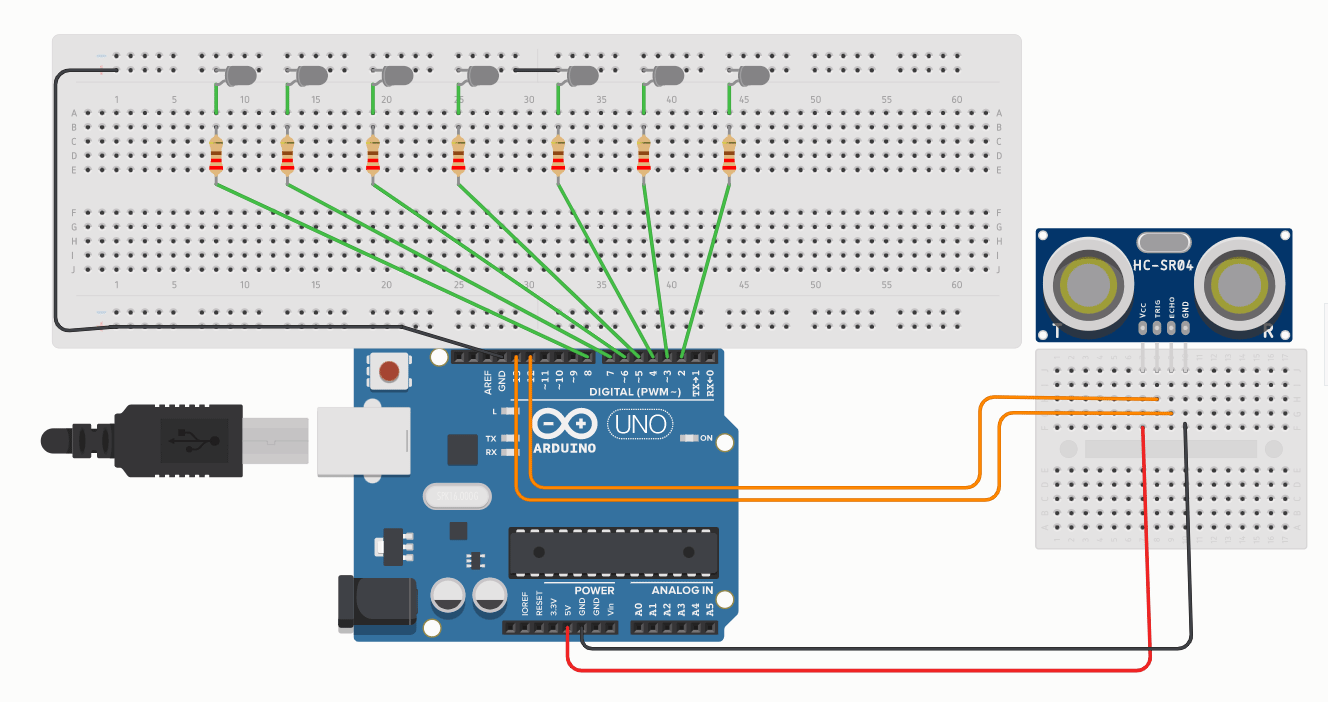## Distance Measuring with LED Notifier using HC-SR04 Ultrasonic Sensor and Arduino

In this tutorial, we are going to learn how to use ultrasonic sensor HC-SR04 interfacing with Arduino and measure the distance which will be notified by 7 LEDs. As we move closer to the sensor the  LED will glow consecutively and vice-versa.

### HC-SR04 Ultrasonic Sensor PinoutThe sensor has 4 pins. 5volts is supplied to the VCC pin and GND is connected to the GND of Arduino. There are two other pins called TRIG and ECHO pins. The Trig pin transmits an ultrasonic wave and the ECHO pin receives the reflected signal from the object.

### HC-SR04 Ultrasonic Sensor Datasheet

 Model No. HC-SR04 Working Voltage 5v Working Current 15mA Working Frequency 40Hz Max Range 4m Min Range 2cm Measuring Angle 15 degree

### HC-SR04 Ultrasonic Sensor Working

Ultrasonic sensors work on the principle that it emits waves at a higher frequency that cannot be heard by humans. Then sensor waits for the wave to be reflected and calculates the distance of how far is the object when in front of the sensor. The practical Distance that can be measured is 2cm to 80cm but theoretically, it is mentioned that the distance can be up to 400 cm.• Arduino IDE

### Circuit Diagram for Distance Measuring LED Notifier### Arduino Code for Distance Measuring LED Notifier

The below code is to check whether the ultrasonic sensor is measuring the distance properly. We can monitor this with the help of a serial monitor on Arduino IDE software. Upload the code and check the serial monitor. It must be showing measuring distance in form of digits.

const int trig = 12;

const int echo = 13;

int duration = 0;

int distance = 0;

void setup() {

pinMode(trig , OUTPUT);

pinMode(echo , INPUT);

Serial.begin(9600);

void loop() {

digitalWrite(trig , HIGH);

delayMicroseconds(1000);

digitalWrite(trig , LOW);

duration = pulseIn(echo , HIGH);

distance = (duration/2) / 29.1 ;

Serial.println(distance);

}

The code below is a complete code for measuring the distance and being notified by LEDs. We are getting the output from pins no 2 to 8 of the Arduino Board which is then connected to LEDs.

const int trig = 12;

const int echo = 13;

const int LED1 = 8;

const int LED2 = 7;

const int LED3 = 6;

const int LED4 = 5;

const int LED5 = 4;

const int LED6 = 3;

const int LED7 = 2;

int duration = 0;

int distance = 0;

void setup() {

pinMode(trig , OUTPUT);

pinMode(echo , INPUT);

pinMode(LED1 , OUTPUT);

pinMode(LED2 , OUTPUT);

pinMode(LED3 , OUTPUT);

pinMode(LED4 , OUTPUT);

pinMode(LED5 , OUTPUT);

pinMode(LED6 , OUTPUT);

pinMode(LED7 , OUTPUT);

Serial.begin(9600);

void loop() {

digitalWrite(trig , HIGH);

delayMicroseconds(1000);

digitalWrite(trig , LOW);

duration = pulseIn(echo , HIGH);

distance = (duration/2) / 28.5 ;

Serial.println(distance);

if ( distance <= 7 )  {

digitalWrite(LED1, HIGH);

}

else {

digitalWrite(LED1, LOW);

}

if ( distance <= 14 ) {

digitalWrite(LED2, HIGH);

}

else {

digitalWrite(LED2, LOW);

}

if ( distance <= 21 ) {

digitalWrite(LED3, HIGH);

}

else {

digitalWrite(LED3, LOW);

}

if ( distance <= 28 ) {

digitalWrite(LED4, HIGH);

}

else {

digitalWrite(LED4, LOW);

}

if ( distance <= 35 ) {

digitalWrite(LED5, HIGH);

}

else {

digitalWrite(LED5, LOW);

}

if ( distance <= 42 ) {

digitalWrite(LED6, HIGH);

}

else {

digitalWrite(LED6, LOW);

}

if ( distance <= 45 ) {

digitalWrite(LED7, HIGH);

}

else {

digitalWrite(LED7, LOW);

}

}

### Ultrasonic sensor Troubleshooting

#### Project not working?

If your project is not working cross-check all your connection as it uses   many connecting wires. Ground wires should be properly connected.

#### One or multiple LED is not glowing?

If your LED is not glowing, there might be a probability that you have used your LED in reverse polarity.

#### Ultrasonic sensor not showing values in serial monitor?

You must have connected the circuit as per the circuit diagram. Have a notice to the four terminals for the ultrasonic sensor.

#### Brightness of the LED is very LOW?

If so, then you must have taken higher a values of resistors. As mentioned you should use value near 220 ohms

#### Want to increase or decrease distance of ultrasonic sensor to glow LED as per your need?

For this, you have to change the values in the code. For example, you need a distance of 35cm then in place of 45cm replace the digits. The rest of the code will remain the same.